Demystifying the Connectionist Temporal Classification Loss

## Background: Speech Recognition Pipelines

Typical speech processing approaches use a deep learning component (either a CNN or an RNN) followed by a mechanism to ensure that there’s consistency in time (traditionally an HMM).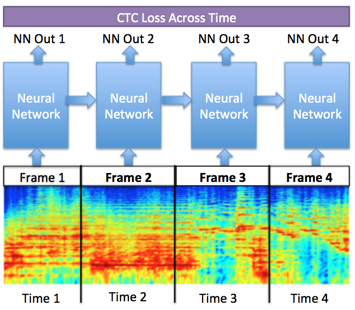• the deep laerning component predicts what’s being uttered, i.e. phoneme, which is frequently based on a single 10ms frame of the input signal. The input signal may be a spectrogram, Mel features, or raw signal. this component is the light blue boxes above.
• the time consistency component deals with rate of speech as well as what’s being said overall. that’s the purple box above.

Since it’s really hard to predict what’s in a 10ms frame without context, this second consistency component is necessary. Its job is to inform our predictions based on previous or future frames. It’s this component that the community has been makeing the wholesale change from HMMs over to RNNs.

Behind the face of RNNs lies a peculiar cost function called the Connectionist Temporal Classification loss, initially presented at ICML in 2006 by Alex Graves and Co. This is quite significant since the HMM(the RNN & CTC predecessor) has been used for speech precoessing since forever, befor and even after neural networks got hot. now let’s find out what does the CTC loss funtion do.

## The Problem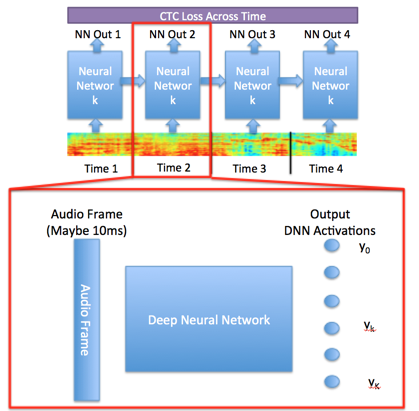Let’s take a closer look Diagram 1 with a blow-up of the neural network in Diagram 2.

At each time step, a neural network predicts an output we call a vector y. Each one of the dimensions of y is an indicator of how much we believe in an utterance given an audio frame. For example, let’s say the 4th dimension of output vector y (denoted by y₄) represents the phoneme “/y/”.

 If y₄ is a value 0.84 and all the other y’s are small (like 0.02), my neural network is pretty sure I’m saying “/y/”. I’m essentially predicting my outputs given my inputs: p( y x ) = Neural-Network( x ), where x is my input frame.

Nothing new, just neural networks 101. But wait, maybe the word I’m saying is, “huge”, and that phoneme was just found near the “/y/” and should really be “/h/”. Unless I’m Donald Trump (I’m not) the “/y/” phoneme is incorrect. In this case, context actually buys us a lot.

Rather than predict each frame at a time, what would be more informative is we predict the sequence of outputs that makes the most sense {y, y, …, y[T]}, where y[t] is a vector with all possible phonemes at time t.

So let’s line up the outputs from Diagram 2 in a row over five time steps, shown in Diagram 3. The connected line through nodes at each time step is called a path, and is often represented by the greek symbol π.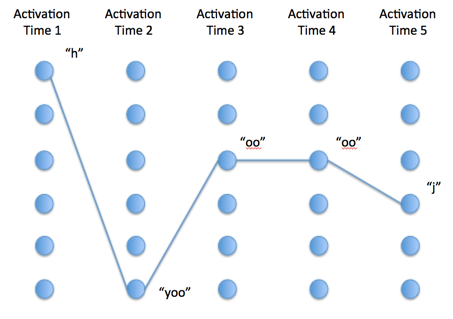The problem is then to optimize for the best path π. In math, you’re optimizing the below equation.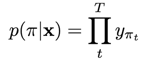That’s just a compact way of saying, the probability of a particular path is the product of all the softmax outputs over time T. Now, you might be wondering, doesn’t recurrence or convolutions take care of that? Just by using a CNN or RNN, you’re already considering past outputs, right?

True, but you might also notice in Diagram 3, “/oo/” appears twice, and doesn’t contribute to the meaning of the phrase. That’s important as rate of speech should be discounted when recognizing words. Instead of “-h-yoo-oo-oo-j-”, we really want “-h-yoo-oo-j-” with the second “oo” deleted.

This is especially noticeable when we consider that silence/blank/repeat /transition characters actually make up a lot of speech. In fact, we’ll often have something that looks like:

— — — bbbb ——eeeee —

which in reality, we should perceive as “be”.

1. Label every single time step, and where the phoneme changes
2. Just do an overall labeling and make the network figure it out

The second option is where the CTC loss comes in.Instead of just optimizing for the path, π, also optimize to the label.

Let’s call that label l. Now, we’re going to predict the best label among all paths, the CTC objective: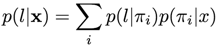The above equation is really just saying, the probability that I’ve said label lis just the probability over the sum of all the possible ways to get there over all paths (i.e., marginalizing out π.) If you want to be fancy, you’d call this step dynamic time warping. If not, just call it getting rid of crap that’s repetitive.

## Solving the Label Problem

Okay, so now we know the problem (i.e., the CTC objective). What’s the solution? (i.e., how do we optimize?) As in the case of HMM’s, we could use dynamic programming. In fact, we can optimize using the analogous forward-backward algorithm.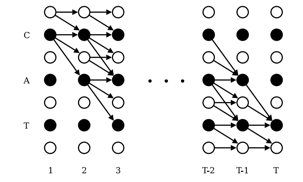Each row in Diagram 4 is a label interspersed with “blanks” or “transitions”, where the entire word is “CAT”. Each column is the time when it’s uttered.

At each time step (again, each column), we’re picking which label could go next, which is demonstrated by the arrows. It could be one of three things:

• My current label
• A transition to the next label
• The next predicted label

Well, how do I choose which one of these things to do? That’s where the forward-backward algorithm comes in.

 There’s some math, but the basic gist of the forward backward algorithm is you’re calculating “p(l x)” with some helper functions “α(t)” and “β(t)”. Going forward, you calculate a parameter “α(t)”. *Going backwards you calculate “β(t)*”, and the probability of a label given your input is: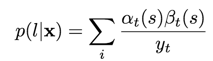Both “α(t)” and “β(t)” are calculated with an input of the two previous equations in this blog (the neural network output y), but you can get them from Graves’ paper. To choose which label comes next, you’re calculating α(t) and *β(t). *The maximum of p(l x) will tell you what phoneme is being uttered.* But more importantly, the key to tractability is you are only considering the paths with “good” labels.* In other words, if you’re not a node with an arrow coming in and going out of you in Diagram 4, then you’re not being considered.

## Final Touches

 But wait. I just said that “α(t)” and “β(t)”* depend on the output of the neural network, y. Doesn’t that mean that when I optimize my neural network, “α(t)”and “β(t)”* will change? Well, yes, but that’s what back propagation is for! We just need to calculate the gradient of “p(l x)” with respect to its input y, which so happens to be what the neural network gives you up until that point. We can do this from the equation that I just described above, but it’s easier just to take equation 15 from Graves in his paper. I’m not going to include it here because if you’re serious about implementation, you’ll probably read his paper anyway. ;-)